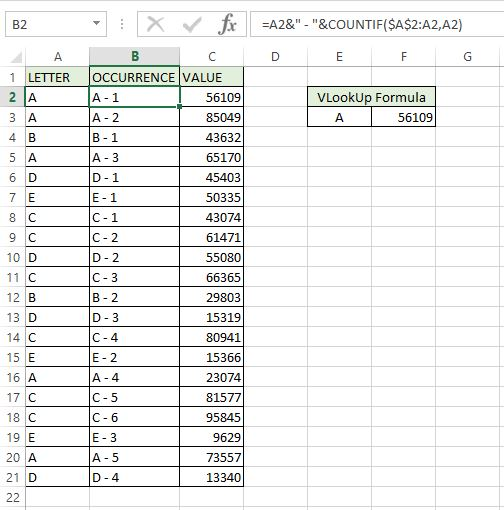# VLookUp To Retrieve data relating to the second or third matchIf you have ever used VLookUps before you may have noticed that the formula only retrieves data relating to the first match it finds.

Did you ever need a VLookUp to retrieve data relating to the second or third match?

In an earlier post called VLookUp with multiple values and  I showed you how to retrieve data for all matches, this time I will show you how to create a VLookUp to retrieve data relating to the second or third match, or any numbered match you choose.

The image below shows a simple VLookUp.  Our look up value is “A” and it retrieves the value relating to the first match of A.What if we wanted to retrieve the value relating to the second, third or even fourth occurrence of A?

We need a way to identify each occurrence of A (and indeed, all our letters in column A) and then we will look up that occurrence.

To add what we will now for want of a better phrase call our occurrence number, we will use the COUNTIF formula.

# COUNTIF

The COUNTIF formula counts how many times our criteria occurs in a given range.

I have added a column B to my existing file.  This is where I will enter the Occurrence Number.

In cell B2, I enter the first COUNTIF as follows

• =COUNTIF(
• \$A\$2:A2 This is the range that we are going to look for our criteria in
• A2) This is our criteria.  We are going to look for the value of A2 in our range and count the number of times it appears.

I then copy this formula down for all rows.

Note the first A has 1 in column B, the second has 2, the third has 3 and so on, the same with each occurence of the remaining letters.How does this work?

The more observant of you may have noticed that the range given is a mixture of absolute and relative references.

Our first cell reference in our range is \$A\$2, this is an absolute reference.  The \$ signs tell Excel not to change the column or row values as we drag the formula down to other rows or across to other columns.  The start of our range will remain as A2.

The second part of the range is A2, this is a relative reference.  When we drag this down a row it becomes A3, then A4 and so on.

Starting in row 2, our range is cell A2 to A2, and “A” only occurs once here.

Then in row 3, our range is cell A2 to A3, “A” occurs twice here.

Then our range is A2 to A4 and “A” still only occurs twice here.

In row 5 our range is A2 to A5 and “A” occurs three times here.

The result of our COUNTIF formula is now the Occurrence Number of each letter.

## Unique Lookup Value

To create a unique value that the VLookUp can use, I have amended the formula in column B.

Before the COUNTIF formula I’ve added in a reference to our letter in column A and then a dash with a space before and after it.

This gives us “A – 1” for the first occurrence of A and then “A – 2” and so on.Now instead of looking up A and hoping against all hope to retrieve the value for the second match, we can look up A – 2 and this will give us the match for the second occurrence of A.

## VLookUp To Retrieve data relating to the second or third match

In Cell G7, I have entered another VLookUp, the lookup value this time is the letter in E7, a dash with spaces and then the occurrence number in F7.

The 3rd occurrence of A has a matching value of 65170.

There are several ways to create the lookup value, this is just one of them.

You can hide column B if you wanted.Is the dash needed?

The short answer is “no”.  Recently when I was working on a file for a team, adding the dashes before the occurrence number made it easier for them to see and quickly identify the occurrence number.

It is clearer to see, so I use it myself now, but it is not a requirement.

To further add to your LookUp arsenal, I will be showing you an alternative to VLookUps in a future post.  Watch out for it.